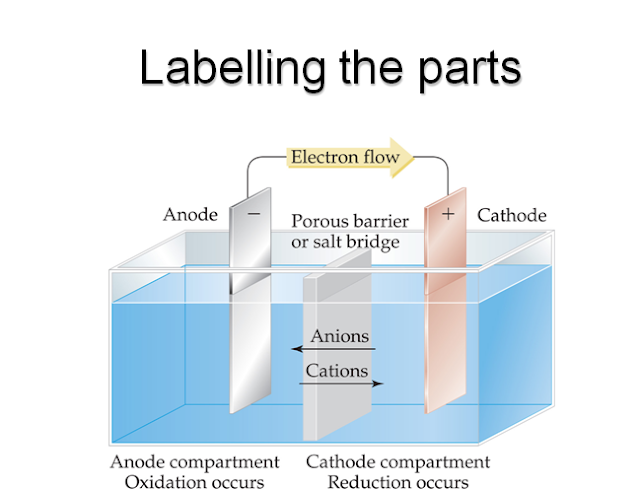SCC Education

Introducation of Electro chemistry

Electro chemistry
Learning objectives
§You will be able to:
§Identify main components of an electrochemical cell
§Write shorthand description of electrochemical cell
§Calculate cell voltage using standard reduction potentials
§Apply Nernst equation to determine free energy change
§Apply Nernst equation to determine pH
§Calculate K from electrode potentials

§Calculate amount of material deposited in electrolysis
Energy in or energy out
§Galvanic (or voltaic) cell relies on spontaneous process to generate a potential
capable of performing work – energy out
§Electrolytic cell performs chemical reactions through application of a potential –
energy in
Redox
§Oxidation is...
§Loss of electrons
§Reduction is...
§Gain of electrons
§Oxidizing agents oxidize and are reduced
§Reducing agents reduce and are oxidized
Redox at the heart of the matter